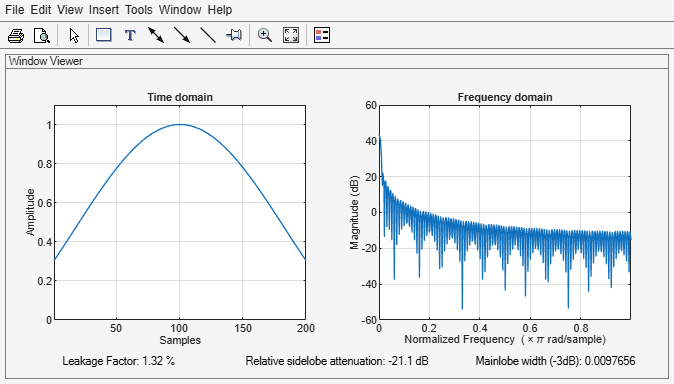# kaiser

## Syntax

w = kaiser(L,beta)

## Description

example

w = kaiser(L,beta) returns an L-point Kaiser window with shape factor beta.

## Examples

collapse all

Create a 200-point Kaiser window with a beta of 2.5. Display the result using wvtool.

w = kaiser(200,2.5); wvtool(w)## Input Arguments

collapse all

Window length, specified as a positive integer.

Data Types: single | double

Shape factor, specified as a positive real scalar. The parameter beta affects the sidelobe attenuation of the Fourier transform of the window.

Data Types: single | double

## Output Arguments

collapse all

Kaiser window, returned as a column vector.

## Algorithms

The coefficients of a Kaiser window are computed from the following equation:

$w\left(n\right)=\frac{{I}_{0}\left(\beta \sqrt{1-{\left(\frac{n-N/2}{N/2}\right)}^{2}}\right)}{{I}_{0}\left(\beta \right)},\text{ }0\le n\le N,$

where I0 is the zeroth-order modified Bessel function of the first kind. The length L = N + 1. kaiser(L,beta) is equivalent to

besseli(0,beta*sqrt(1-(((0:L-1)-(L-1)/2)/((L-1)/2)).^2))/besseli(0,beta)

To obtain a Kaiser window that represents an FIR filter with sidelobe attenuation of α dB, use the following β.

$\beta =\left\{\begin{array}{ll}0.1102\left(\alpha -8.7\right),\hfill & \alpha >50\hfill \\ 0.5842{\left(\alpha -21\right)}^{0.4}+0.07886\left(\alpha -21\right),\hfill & 50\ge \alpha \ge 21\hfill \\ 0,\hfill & \alpha <21\hfill \end{array}$

Increasing β widens the mainlobe and decreases the amplitude of the sidelobes (i.e., increases the attenuation).

 Digital Signal Processing Committee of the IEEE Acoustics, Speech, and Signal Processing Society, eds. Selected Papers in Digital Signal Processing. Vol. II. New York: IEEE Press, 1976.

 Kaiser, James F. "Nonrecursive Digital Filter Design Using the I0-Sinh Window Function." Proceedings of the 1974 IEEE® International Symposium on Circuits and Systems. April, 1974, pp. 20–23.

 Oppenheim, Alan V., Ronald W. Schafer, and John R. Buck. Discrete-Time Signal Processing. Upper Saddle River, NJ: Prentice Hall, 1999.